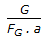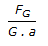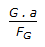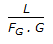# Chemical Engineering - Mass Transfer

11.

At 750°K and 1 atm, the approximate value of Schmidt number for air is

 A. 0.01 B. 0.1 C. 1 D. 10

Explanation:

No answer description available for this question. Let us discuss.

12.

Calculation of mass transfer co-efficient is mostly/normally done using __________ theory.

 A. surface renewal B. film C. penetration D. none of these.

Explanation:

No answer description available for this question. Let us discuss.

13.

The velocity, concentration and temperature boundary, for the boundary layer development on a flat plate during connective mass transfer will be same, if

 A. NSc = 1 B. NSc = NPr = NLe C. NPr = NLe D. NSc = NLe

Explanation:

No answer description available for this question. Let us discuss.

14.

For the gas absorption, the height of a transfer unit, based on the gas phase is given by (G: superficial molar gas velocity, L: superficial molar liquid velocity, FG:mass transfer co-efficient, mols/m2 , a: in-terfacial area per unit volume of tower)

 A.B.C.D.Explanation:

No answer description available for this question. Let us discuss.

15.

Which of the following is used for the concentration of rubber latex ?

 A. Agitated film evaporator B. Long tube vertical evaporator C. Short tube evaporator D. Calendria